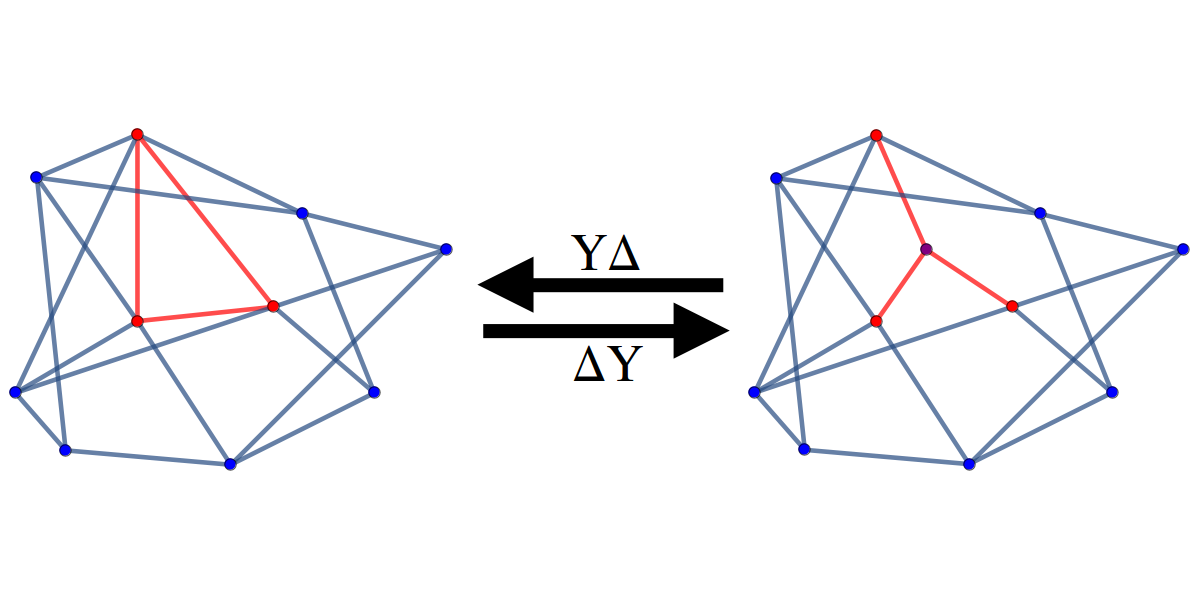{{ message }}

# mikepierce / YTYGraphTransforms

Mathematica package for performing triangle-wye (TY) and wye-triangle (YT) transforms

## Files

Failed to load latest commit information.
Type
Name
Commit time

# Wye-Triangle-Wye Graph TransformsA Mathematica package that contains functions for performing triangle-wye (also delta-wye or ΔY) and wye-triangle (also wye-delta or YΔ) transforms on simple undirected graphs.The main feature of this package is the function `WyeTriangleWyeFamily` that can efficiently generate all (or a selection of) graphs that are the result of any sequence of triangle-wye or wye-triangle transforms on a graph.

# Functions

• `TriangleWye[g, {a,b,c}]` performs a triangle-wye transform on the triangle given by vertices {a, b, c} in graph g by deleting any edges among the vertices {a, b, c} and adding a new vertex incident to each of {a, b, c}.

• `TriangleWyeChildren[g]` returns every graph (distinct up to isomorphism) that can be created by performing a triangle-wye transform on some triangle in g. Relies on function `TriangleWye`.

• `TriangleWyeDescendants[g]` returns a nested list of all (not necessarily distinct) graphs that can be created by performing a sequence of triangle-wye transforms on g. The nested lists keep track of the family heritage and looks like `{graph, TriangleWyeDescendants /@ TriangleWyeChildren[graph]}`. Relies on function `TriangleWyeChildren`.

To get a flat list of the distinct triangle-wye descendants of g, use

`````` DeleteDuplicates[Flatten[TriangleWyeDescendants[g]], IsomorphicGraphQ[#1, #2] &]
``````
• `WyeTriangle[g, v]` performs a wye-triangle transform on vertex v in graph g by deleting v and forming a 3-cycle among the neighbors of v. This function fails if `VertexDegree[g, v] != 3`.

• `WyeTriangleParents[g]` returns every graph (distinct up to isomorphism) that can be created by performing a wye-triangle transform on some degree 3 vertex in g. Relies on function `WyeTriangle`.

`WyeTriangleParents[g, True]` does the same thing using a stricter definition of a wye-triangle transform where the neighbors of the vertex to be deleted must not be neighbors of each other.

• `WyeTriangleAncestors[g]` returns a nested list of all (not necessarily distinct) graphs that can be created by performing a sequence of wye-triangle transforms on g. The nested lists keep track of the family heritage and looks like `{graph, WyeTriangleAncestors /@ WyeTriangleParents[graph]}`. Relies on function `WyeTriangleParents`.

`WyeTriangleAncestors[g, True]` does the same thing using a stricter definition of a wye-triangle transform where the neighbors of the vertex to be deleted must not be neighbors of each other.

To get a flat list of the distinct wye-triangle ancestors of g, use

`````` DeleteDuplicates[Flatten[WyeTriangleAncestors[g]], IsomorphicGraphQ[#1, #2] &]
``````
• `WyeTriangleWyeFamily[g]` returns a flattened list of all distinct graphs that can be created by performing a sequence of triangle-wye and wye-triangle transforms on g, where g is either a single graph or a list graphs. Relies on functions `WyeTriangleParents` and `TriangleWyeChildren`.

`WyeTriangleWyeFamily[g, Limit, ProgressFlag, LogFlag, Strict, Condition]` returns a flattened list of all distinct graphs that can be created by performing a sequence of triangle-wye and wye-triangle transforms on g with a few optional conditions:

• Limit is a positive integer such that the function will produce only the graphs that are no more than Limit triangle-wye and wye-triangle transforms from g.

• Set ProgressFlag to `True` to print some output to indicate the progress of the algorithm. In this output, `G` is the starting graph (or set of graphs), and each row contains the list of (the sizes of) sets of graphs produced with the same edge count.

• Set LogFlag to `True` to produce a simple log file containing the edgelists of the graphs produced.

• Set Strict to `True` to use a stricter definition of a wye-triangle transform where the neighbors of the vertex to be deleted must not be neighbors of each other.

• Condition should be a function that takes a single graph object and returns `True` or `False`. If Condition is defined, all graphs (except those of g) that don't satisfy Condition will be immediately discarded from consideration. By default, Condition is `GraphQ`.

# ToDo

• Change the strictness second argument in `WyeTriangleParents` and `WyeTriangleAncestors` and all the arguments in `WyeTriangleWyeFamily` to utilize `OptionsPattern[]` and `OptionsValue[]`.
• Add a feature to `WyeTriangleWyeFamily` (and maybe to the Ancestor/Descendent functions too) to produce a graphic of the family showing the lineage.

Mathematica package for performing triangle-wye (TY) and wye-triangle (YT) transforms